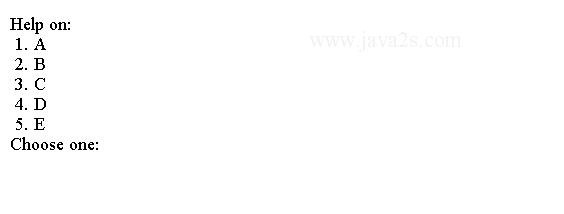# Java Tutorial - Java While

The `while` loop repeats a statement or block while its controlling `condition` is `true`.

## Java while Loop

Here is its general form:

```while(condition) {
// body of loop
}
```
• The `condition` can be any `Boolean` expression.
• The body of the loop will be executed as long as the conditional `condition` is `true`.
• The curly braces are unnecessary if only a single statement is being repeated.

Here is a while loop that counts down from 10, printing exactly ten lines of "tick":

```
public class Main {
public static void main(String args[]) {
int n = 10;
while (n > 0) {
System.out.println("n:" + n);
n--;
}
}
}
```

When you run this program, you will get the following result: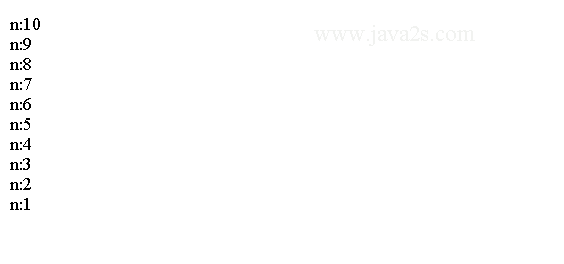## Example

The following code shows how to use the while loop to calculate sum.

```public class Main {
public static void main(String[] args) {
int limit = 20;
int sum = 0;/*from   ww  w.j a  va 2  s. c om*/
int i = 1;

while (i <= limit) {
sum += i++;
}
System.out.println("sum = " + sum);
}
}
```

The code above generates the following result.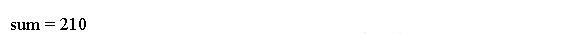## Example 2

The body of the `while` loop will not execute if the condition is `false`. For example, in the following fragment, the call to `println()` is never executed:

```
public class Main {
public static void main(String[] argv) {
int a = 10, b = 20;
while (a > b) {
System.out.println("This will not be displayed");
}
System.out.println("You are here");
}
}
```

The output: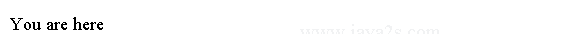## Example 3

The body of the `while` can be empty. For example, consider the following program:

```
public class Main {
public static void main(String args[]) {
int i, j;//from  w ww. ja v a  2  s . co  m
i = 10;
j = 20;

// find midpoint between i and j
while (++i < --j)
;
System.out.println("Midpoint is " + i);
}
}
```

The `while` loop in the code above has no loop body and `i` and `j` are calculated in the while loop condition statement. It generates the following output: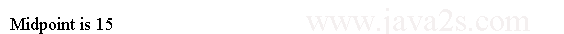## Java do while loop

To execute the body of a while loop at least once, you can use the do-while loop.

The syntax for Java do while loop is:

```do {
// body of loop
} while (condition);
```

Here is an example to show how to use a `do-while` loop.

```
public class Main {
public static void main(String args[]) {
int n = 10;
do {
System.out.println("n:" + n);
n--;
} while (n > 0);
}
}
```

The output: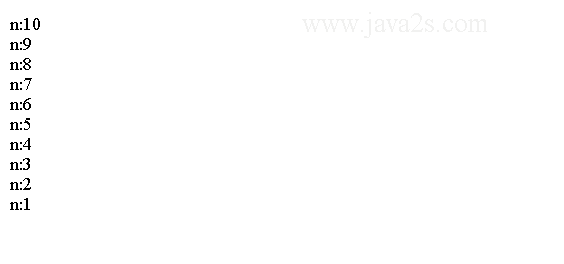The loop in the preceding program can be written as follows:

```public class Main {
public static void main(String args[]) {
int n = 10;
do {
System.out.println("n:" + n);
} while (--n > 0);
}
}
```

The output is identical the result above:## Example 4

The following program implements a very simple help system with `do-while` loop and `switch` statement.

```
public class Main {
public static void main(String args[]) throws java.io.IOException {
char choice;// ww  w.  j  av  a  2  s .  c  om
do {
System.out.println("Help on:");
System.out.println(" 1. A");
System.out.println(" 2. B");
System.out.println(" 3. C");
System.out.println(" 4. D");
System.out.println(" 5. E");
System.out.println("Choose one:");
} while (choice < '1' || choice > '5');
System.out.println("\n");
switch (choice) {
case '1':
System.out.println("A");
break;
case '2':
System.out.println("B");
break;
case '3':
System.out.println("C");
break;
case '4':
System.out.println("D");
break;
case '5':
System.out.println("E");
break;
}
}
}
```

Here is a sample run produced by this program: